To start off the blog, I've chosen the most basic example I could come up with:

Medical imaging categorization based on comparison between the "statistically average" image from a category and a set of test images.

This will be used to build upon using more advanced techniques, so stay tuned!

! rm -rf ./medical_mnist
! git clone https://github.com/apolanco3225/Medical-MNIST-Classification.git
! mv Medical-MNIST-Classification/resized/ ./medical_mnist
! rm -rf Medical-MNIST-Classification

Cloning into 'Medical-MNIST-Classification'...
remote: Enumerating objects: 58532, done.
remote: Total 58532 (delta 0), reused 0 (delta 0), pack-reused 58532
Receiving objects: 100% (58532/58532), 77.86 MiB | 4.39 MiB/s, done.
Resolving deltas: 100% (506/506), done.
Checking connectivity... done.
Checking out files: 100% (58959/58959), done.


install useful libraries

# !pip install torch torchvision


data will be downloaded to medical_mnist folder

from pathlib import Path

data = Path('medical_mnist')
list(data.iterdir())

[PosixPath('medical_mnist/AbdomenCT'),
PosixPath('medical_mnist/BreastMRI'),
PosixPath('medical_mnist/CXR'),
PosixPath('medical_mnist/ChestCT'),
PosixPath('medical_mnist/Hand'),
PosixPath('medical_mnist/HeadCT')]

let's see what we have here... as this is the most basic technique, let's pick the images that look the most different from each other

import matplotlib.pyplot as plt
from PIL import Image

for d in data.iterdir():
print(d)
plt.imshow(Image.open(list(d.iterdir())))
plt.show()

medical_mnist/AbdomenCT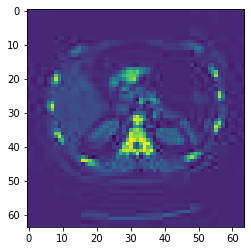medical_mnist/BreastMRI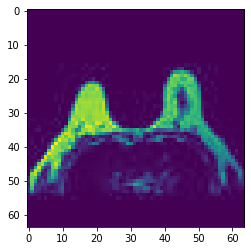medical_mnist/CXR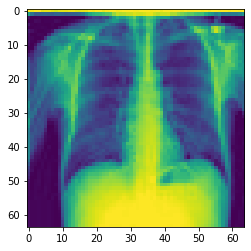medical_mnist/ChestCT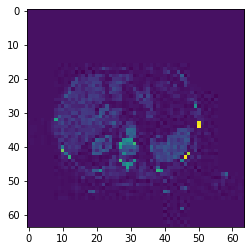medical_mnist/Hand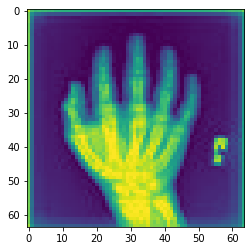medical_mnist/HeadCT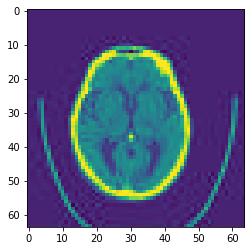import torch
from torchvision.transforms import ToTensor

stacked_cxrs = torch.stack([ToTensor()(Image.open(path)).float()/255 for path in (data/'CXR').iterdir()])


as a good practice, let's look at the first image, so see if we did it correctly

plt.imshow(stacked_cxrs)

<matplotlib.image.AxesImage at 0x7f2198995610>now, let's build "ideal" image for each of the category. This ideal image is just a mean for each pixel across all the images

mean_cxrs = stacked_cxrs.mean(0)

plt.imshow(mean_cxrs)

<matplotlib.image.AxesImage at 0x7f21717d1590>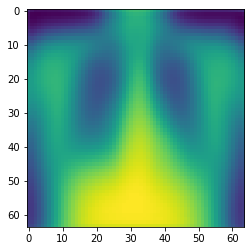mean_headct = stacked_heads.mean(0)


<matplotlib.image.AxesImage at 0x7f211f6b6a50>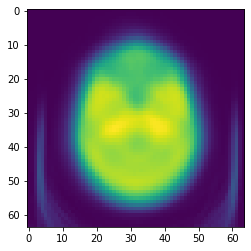now we can see how much example image differs from the ideals:

import torch.nn.functional as F

F.mse_loss(stacked_cxrs, mean_cxrs).sqrt()

tensor(0.0010)
F.mse_loss(stacked_cxrs, mean_headct).sqrt()

tensor(0.0016)

looks like that one was a CXR indeed - L2 loss between ideal image from CXR category (mean_cxrs) was lower

so let's build a simple classifier function, that predicts whether image is a headct or not

def is_headct(img_tensor):
if F.mse_loss(img_tensor, mean_cxrs) > F.mse_loss(img_tensor, mean_headct):
return True
else:
return False


now we test the classifier

cxrs_preds = torch.tensor([not is_headct(stacked_cxrs[i]) for i in range(stacked_cxrs.shape)])
cxrs_accuracy = cxrs_preds.sum().float() / cxrs_preds.shape
print(f'Accuracy on CXRs: {round( (cxrs_accuracy).item() * 100, 2)}%')

Accuracy on CXRs: 99.15%

head_preds = torch.tensor([is_headct(stacked_heads[i]) for i in range(stacked_heads.shape)])

Accuracy on HeadCTs: 100.0%

2. we didn't split into train and test sets here so results are biased (as each image we predict was used to figure out the "ideal" image)